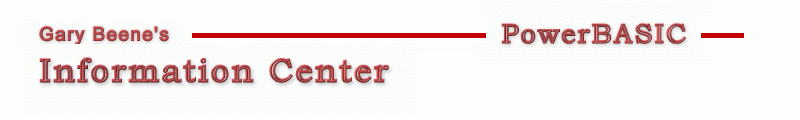## Placement

Category: Utilities

Date: 03-28-2012

'This code was written to compilete in PBWin10. To compile with PBWin9,
'replace CALL with USING in Array Sort (2 places in code below)

'Primary Code:
Sub PlaceParts_Std
'left to right, then down when xMax is exceeded. as the parts are found in the list
Local i,x,y,xk,yk,xedge,yedge,xcenter,ycenter,xdelta,ydelta As Long
For i = 1 To UBound(PL)
x = PL(i).x + 2*PL(i).xk : y = PL(i).y + 2*PL(i).yk  'convenience variables, used in this function
Select Case (xedge+x)
Case < xMax     'keep moving right
PL(i).xcenter = xedge + x/2 : PL(i).ycenter = yedge + y/2
If ydelta < y Then ydelta = y
xedge = xedge + x
Case = xMax     'draw here, then next part at left
PL(i).xcenter = xedge + x/2 : PL(i).ycenter = yedge + y/2
If ydelta < y Then ydelta = y
yedge = yedge + ydelta : ydelta = 0 : xedge = 0
Case > xMax     'draw this part at left
yedge = yedge + ydelta
PL(i).xcenter = x/2 : PL(i).ycenter = yedge + y/2
ydelta = y : xedge = x
End Select
DrawPart(i)                  'draw the part (part body + keepout zone)
Next i
DrawContainer                   'bounding rectangle
End Sub

'Compilable Example:
#Compiler PBWin 10
#Compile EXE
#Dim All
#Debug Error On     'catch array/pointer errors - OFF in production
#Debug Display On   'display untrapped errors   - OFF in production#Include "Win32API.inc"

Type PartType
x As Long
y As Long
z As Long
xk As Long
yk As Long
xcenter As Long
ycenter As Long
area As Long
placed As Long
End Type

Global hDlg As DWord
Global PL(), PLMin() As PartType   'PartList
Global xRequired,yRequired,xMax,yMax,GapFiller As Long

Function PBMain() As Long
Dialog New Pixels, 0, "Placement Demo",300,300,750,460, %WS_OverlappedWindow To hDlg
Control Add Button,  hDlg, 100,"Place_Std", 130,30,100,20
Control Add Button,  hDlg, 101,"Place_Sort", 130,60,100,20
Control Add Button,  hDlg, 102,"Place_Rnd", 130,90,100,20
Control Add Button,  hDlg, 103,"Place_Opt", 130,120,100,20

Control Add TextBox, hDlg, 201,"20 20 30 20", 10,25,100,20
Control Add TextBox, hDlg, 202,"120 40 30 5", 10,50,100,20
Control Add TextBox, hDlg, 203,"40 40 50 5", 10,75,100,20
Control Add TextBox, hDlg, 204,"80 30 80 5", 10,100,100,20

Control Add Label,   hDlg, 501,"Max x-Size:",10,130,100,15
Control Add TextBox, hDlg, 200,"400 400", 10,145,100,20
Control Add ListBox, hDlg, 400,, 10,195,200,275, %WS_TabStop Or %WS_Vscroll,%WS_Ex_ClientEdge

Control Add Graphic, hDlg, 300, "", 270,20,800,800
Graphic Attach hDlg, 300
Dialog Show Modal hDlg Call DlgProc
End Function

CallBack Function DlgProc() As Long
Local i,j,k,m,iLoops,RequiredArea,RequiredX,RequiredY As Long, temp As PartType
Select Case CB.Msg
Case %WM_Command
Select Case CB.Ctl
Case 100
Graphic Clear
PlaceParts_Std
DisplayInfo
Case 101
Graphic Clear
Array Sort PL(1), Call CustomSortDescend   'sort in descending order
PlaceParts_Std
DisplayInfo
Case 102
Randomize Timer
RequiredArea = 99999999     'arbitrarily large starting point
ReDim PLMin(UBound(PL))
Control Get Check hDlg, 104 To iLoops : iLoops = 1 + iLoops*99   '1 or 100
For k = 1 to iLoops            'iterate placement
Graphic Clear
For i = 1 to UBound(PL)
j = Rnd(1,UBound(PL)) :  Swap PL(i),PL(j)      'random swap
Next i
PlaceParts_Std
'keep the results requiring the smallest area
If RequiredArea > (xrequired*yrequired) Then
RequiredArea = xrequired*yrequired                   'area
RequiredX = xrequired : RequiredY = yrequired        'dimensions
For M = 0 TO UBound(PL) : PLMin(M) = PL(M) : Next M  'part layout
End If
Next k
For M = 0 TO UBound(PL) : PL(M) = PLMin(M) : Next M        'restore the minimum layout
Graphic Clear
For i = 1 to UBound(PL) : DrawPart(i) : Next i             'draw minimum layhout
xrequired = RequiredX  :  yrequired = RequiredY            'restore required dimensions
DrawContainer
DisplayInfo
Case 103
Graphic Clear
Array Sort PL(1), Call CustomSortDescend   'sort in descending order
GapFiller = 1 : PlaceParts_Std : GapFiller = 0
DisplayInfo
End Select
End Select
End Function

Local temp As String, i,j,pCount As Long

ReDim PL(0)          'zero element not used
'perimeter
Control Get Text hDlg, 200 To temp
xMax = Val(Parse\$(temp," ",1))
yMax = Val(Parse\$(temp," ",2))

'part dimensions and keepouts
For i = 201 To 204
Control Get Text hDlg, i To temp
pCount = Val(Parse\$(temp, " ", 4))
ReDim Preserve PL(UBound(PL)+pCount)
For j = UBound(PL)- pCount+1 To UBound(PL)
PL(j).x =   Val(Parse\$(temp, " ", 1))
PL(j).y =   Val(Parse\$(temp, " ", 2))
PL(j).z =   Val(Parse\$(temp, " ", 3))
PL(j).xk = 10
PL(j).yk = 10
PL(j).area = (PL(j).x+PL(j).xk) * (PL(j).y+PL(j).yk)
Next i
Next i
End Sub

Sub PlaceParts_Std
'left to right, then down when xMax is exceeded. as the parts are found in the list
Local i,x,y,xk,yk,xedge,yedge,xcenter,ycenter,xdelta,ydelta As Long
xrequired = 0 : yrequired = 0 : For i = 1 to UBound(PL) : PL(i).placed = 0 : Next i  'initialize
For i = 1 To UBound(PL)
If PL(i).placed = 1 Then Iterate For Else PL(i).placed = 1
x = PL(i).x + 2*PL(i).xk : y = PL(i).y + 2*PL(i).yk  'convenience variables, used in this function
Select Case (xedge+x)
Case < xMax     'keep moving right
xRequired = Max (xedge+x,xRequired)
PL(i).xcenter = xedge + x/2 : PL(i).ycenter = yedge + y/2
If ydelta < y Then ydelta = y
xedge = xedge + x
Case = xMax     'draw here, then next part at left
xRequired = Max (xMax,xRequired)
PL(i).xcenter = xedge + x/2 : PL(i).ycenter = yedge + y/2
If ydelta < y Then ydelta = y
yedge = yedge + ydelta
ydelta = 0
xedge = 0
Case > xMax     'draw this part at left
xRequired = Max (xedge,xRequired)
If GapFiller Then FillGap i,xedge,yedge,ydelta
yedge = yedge + ydelta
PL(i).xcenter = x/2 : PL(i).ycenter = yedge + y/2
ydelta = y
xedge = x
End Select
yRequired = yedge + ydelta
DrawPart(i)                  'draw one part at a time
Next i
DrawContainer
End Sub

Sub DrawContainer
Graphic Style 2
Graphic Box (0,0)-(xrequired,yrequired),0,%Red,-2,0
End Sub

Sub DrawPart(i As Long)
Local x1,x2,y1,y2 As Long
x1 = PL(i).xcenter - PL(i).x/2
x2 = PL(i).xcenter + PL(i).x/2
y1 = PL(i).ycenter - PL(i).y/2
y2 = PL(i).ycenter + PL(i).y/2
Graphic Style 0
Graphic Box (x1,y1)-(x2,y2),0,%Blue,-2,0
Graphic Style 2
Graphic Box (x1-PL(i).xk,y1-PL(i).yk)-(x2+PL(i).xk,y2+PL(i).yk),0,%Blue,-2,0
Graphic Set Pos (PL(i).xcenter,PL(i).ycenter)
Graphic Print Str\$(i)
End Sub

Sub DisplayInfo
Local i As Long, temp As String, PartArea, PartPlusArea, eff As Single
ListBox Reset hDlg, 400
ListBox Add hDlg, 400, "Parts: " + Str\$(UBound(PL))
ListBox Add hDlg, 400, "Required Area (x*y): " + Format\$(xRequired*yRequired,"#,000,000")
ListBox Add hDlg, 400, "Required Size (xy):  " + Str\$(xRequired) + "x" + Str\$(yRequired)
ListBox Add hDlg, 400, "Parts List:  x-y-z-xcenter-ycenter-area"
For i = 1 To UBound(PL)
PartArea = PartArea + PL(i).x*PL(i).y
PartPlusArea = PartPlusArea + PL(i).area
temp = Str\$(i) + " "+ Str\$(PL(i).x) + " " + Str\$(PL(i).y) + " " + Str\$(PL(i).z) + " " _
+ Str\$(PL(i).xcenter) + " " + Str\$(PL(i).ycenter) + Str\$(PL(i).x*PL(i).y)
Next i
ListBox Insert hDlg, 400, 4, "Efficiency: " + Format\$(PartArea/(xrequired*yrequired)*100,"0")+"%" + "  w/o keepout"
ListBox Insert hDlg, 400, 5, "Efficiency+: " + Format\$(PartPlusArea/(xrequired*yrequired)*100,"0")+"%" + "  w/keepout
End Sub

Function CustomSortAscend(R As PartType, S As PartType) As Long
If R.area < S.area Then Function = -1 : Exit Function
If R.area > S.area Then Function = +1 : Exit Function
End Function

Function CustomSortDescend(R As PartType, S As PartType) As Long
If R.area > S.area Then Function = -1 : Exit Function
If R.area < S.area Then Function = +1 : Exit Function
End Function

Sub FillGap(i As Long, xe As Long, ye As Long, yd As Long)
Local gxMax,gyMax,j,x,y As Long, temp As String
gxMax = xMax - xe
gyMax = yd
For j = UBound(PL) To i+1 Step - 1
If gxMax <= 0 Then Exit For
If PL(j).placed = 1 Then Iterate For
If ((PL(j).x+PL(j).xk) <= gxMax) AND ((PL(j).y+PL(j).yk)<=gyMax) Then
'set part properties
PL(j).xcenter = xe + (PL(j).x+2*PL(j).xk)/2 : PL(j).ycenter = ye + (PL(j).y+2*PL(j).yk)/2
PL(j).placed = 1
DrawPart j
'reduce gapsize (x-only)
gxMax = gxMax - PL(j).x - 2*PL(j).xk
xe = xe + PL(j).x + 2*PL(j).xk
xRequired = Max(xe,xRequired)
temp = temp + Str\$(j)
End If
Next i
End Sub

'gbs_00577
'Date: 03-10-2012

created by gbSnippets
http://www.garybeene.com/sw/gbsnippets.htm